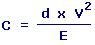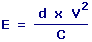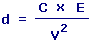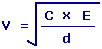Cauchy Number Calculators Enter value, select unit and click on calculate. Result will be displayed.

Calculate Cauchy Number
Calculate Bulk Modulus Elasticity
Calculate Density
Calculate Flow Velocity

 Web www.calculatoredge.com

Calculate Cauchy NumberC = Cauchy Number d = Density V = Velocity E = Bulk Modulus Elasticity
 Enter your values: Flow Velocity: Meter / Second Foot / Second Centimeter / Hour Centimeter / Second Foot / Day Inch / Hour Inch / Second Kilometer / Second Knot Meter / Day Mile / Hour Mile / Second Millimeter / Second Yard / Second Density: Kilogram / Meter^3 Gram / Centimeter^3 Pound / Foot^3 Gram / Meter^3 Milligram / Liter Bulk Modulus Elasticity: Newton/Meter^2 Pascal Atmosphere Bar Dyne/Centimeter^2 Foot of Water Inch of Mercury Inch of Water Kilonewton/Meter^2 Millibar Pound/Foot^2 Pound/Inch^2 Torr Result: Cauchy Number:

Calculate Bulk Modulus ElasticityE = Bulk Modulus Elasticity d = Density V = Velocity C = Cauchy Number
 Enter your values: Flow Velocity: Meter / Second Foot / Second Centimeter / Hour Centimeter / Second Foot / Day Inch / Hour Inch / Second Kilometer / Second Knot Meter / Day Mile / Hour Mile / Second Millimeter / Second Yard / Second Density: Kilogram / Meter^3 Gram / Centimeter^3 Pound / Foot^3 Gram / Meter^3 Milligram / Liter Cauchy Number: Result: Bulk Modulus Elasticity: Newton/Meter2

Calculate Densityd = Density C = Cauchy Number E = Bulk Modulus Elasticity V = Velocity
 Enter your values: Cauchy Number: Bulk Modulus Elasticity: Newton/Meter^2 Pascal Atmosphere Bar Dyne/Centimeter^2 Foot of Water Inch of Mercury Inch of Water Kilonewton/Meter^2 Millibar Pound/Foot^2 Pound/Inch^2 Torr Flow Velocity: Meter / Second Foot / Second Centimeter / Hour Centimeter / Second Foot / Day Inch / Hour Inch / Second Kilometer / Second Knot Meter / Day Mile / Hour Mile / Second Millimeter / Second Yard / Second Result: Density: Kilogram/Meter3

Calculate Flow VelocityV = Velocity C = Cauchy Number E = Bulk Modulus Elasticity d = Density
 Enter your values: Cauchy Number: Bulk Modulus Elasticity: Newton/Meter^2 Pascal Atmosphere Bar Dyne/Centimeter^2 Foot of Water Inch of Mercury Inch of Water Kilonewton/Meter^2 Millibar Pound/Foot^2 Pound/Inch^2 Torr Density: Kilogram / Meter^3 Gram / Centimeter^3 Pound / Foot^3 Gram / Meter^3 Milligram / Liter Result: Flow Velocity: Meter/SecondLINKSDISCLAIMERCONTACT US